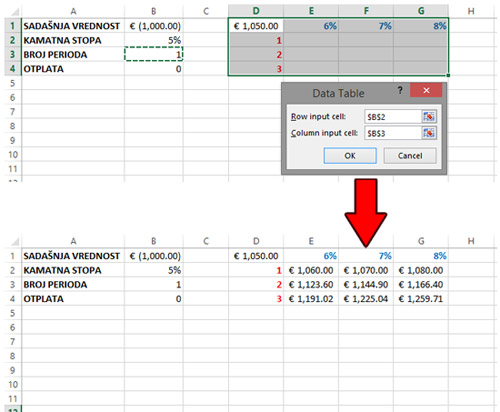# Data tables

One of the What-If analysis tools are Data tables. It is an option that allows the formula’s arguments to be taken from the rows or columns of a specially formed table and thus automatically populated. In practice, this option is not used as often, but it can be handy when we use functions that have more arguments to see the value of the results depending on the change of arguments.

Let’s demonstrate the use of the data table on the example of the FV function to calculate the future value of the investment. To begin with, we will introduce several variables in order to make a formula in which the future value will be calculated. In cells B1 through B4, we enter in the order: the present value, the value of the interest rate, the number of periods and repayment. Then we will enter the formula in D2:

=FV(B2,B3,B4,B1)

Using this formula, we calculate the future value for the invested € 1000, with an interest rate of 5%, for one year without repayment.

Imagine that we now want to form a table in which, for different interest rates, the future investment values ​​would be automatically calculated. First we need to enter the initial rate of interest rate (5%) above the formula and then to the right of it several values ​​we want to calculate alternative values ​​(6%, 7%, 8%). Then we need to select the area that contains both rows: upper, with interest rates, as well as lower, with formulas and empty cells where alternative values ​​of future investments should be calculated. Once we are positioned on the Data ribbon, and choosing the Data Table from the What-If Analysis menu, a dialog box will appear on the screen, where two values ​​can be entered: the Row Input Cell and the Column Input Cell. Depending on where alternative values ​​are found, we enter the address in one of the two fields described. In our example, as the alternative interest rates are in rows, we will increase the value of the Row Input Cell. What do we enter? Enter the address of the cell to which the formula is referenced and whose value should be replaced by alternative values ​​from the table. In our example, it is cell \$B\$2 because it is referenced by the formula in cell D2. After we confirm the listing, the alternative value of the future investment will automatically be filled.We have just shown an example in which the future value of an account is only based on the change of parameters in a row. What would happen if some other value changed. For example, if we want to see a change in the future value depending on the change in the interest rate and the number of years of funding. In this case, the initial table is formed in a slightly different way: in the rows, interest rates can remain, and in the columns we will indicate the years of financing. On the cross-section of rows and columns there will be a formula for calculating the future value. We will select an area containing the formula, rows, columns and places where alternative values should be calculated. Then we repeat the procedure: in the Data ribbon, from the What-If Analysis menu we select the Data Table option. In the dialog window that appears in the Row Input Cell field, we enter \$B\$2 and in the Column Input Cell \$B\$3. Once we confirm the entry, the alternative values will automatically be calculated.